## 5.4Residual

In Sec. 5.3 , we established a criterion for convergence of the Gauss-Seidel method. We now need a way to estimate a level of convergence to determine when to stop iterating.

The analysis of convergence centred on the solution error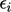, introduced in Sec. 5.2 . In practice,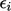cannot be determined since the exact solution is unknown. Instead the residual provides a measure of the accuracy of the solution. The residual vector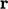represents the change to the solution of the equation, required to make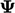exact, according to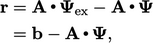(5.10)
where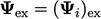.
For the remainder of this chapter, the matrix notation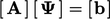is replaced by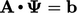, equivalent to vector notation with an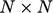tensor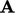.

The vector(of size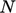) provides one value per matrix row, with both positive and negative values. A measure of residual given by a single value, is deﬁned as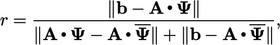(5.11)
where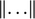is the matrix norm, calculated as the sum of the magnitude of each component, e.g.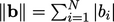; the mean value of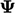over all cells is denoted by.

The residual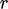provides a measure of error in the solution of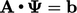, rather than the absolute error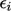. It is divided by the norms of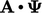and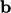to reduce its dependency on the scale of the geometry and solution variable. By reducing its scale-dependency,can be used to compare the level of error equitably between simulations at diﬀerent scales.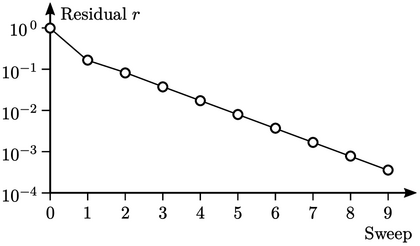The ﬁgure above shows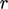calculated from Eq. (5.11 ) following successive sweeps of the Gauss-Seidel method (starting from the initial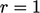). The graph uses a logarithmic vertical scale since the values ofextend over 4 orders of magnitude.

### Tolerance

CFD software generally provides the following controls to stop the iterative solver:

• absolute tolerance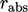;
• relative tolerance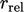.

Sweeping ceases if either tolerance condition is satisﬁed: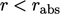; or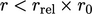, where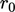is the initial residual within the particular solution step. Thecriterion is often deactivated by setting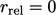, especially for transient simulations when suﬃcient accuracy is required at every solution step.

Notes on CFD: General Principles - 5.4 Residual